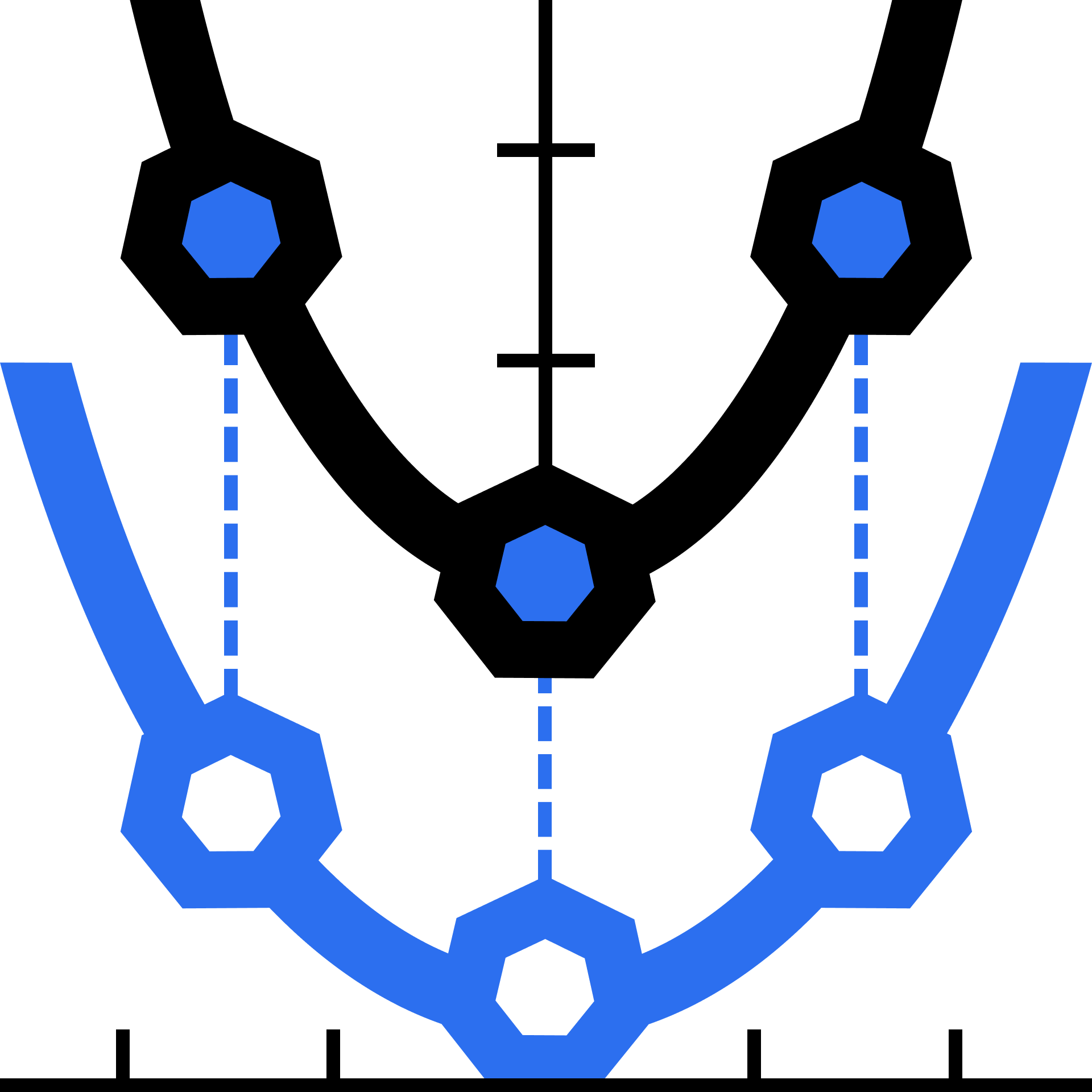Foundational Math · Level 4

Learn about functions and how to work with them. Then, take a deep dive into quadratics.

What is a Function?

Tables and Graphs

Domain and Range

Rigid Transformations

Vertical Stretch and Shrink

Horizontal Stretch and Shrink

Transforming Parabolas

Factoring Special Cases

Completing the Square

The General Solution

The Discriminant

### Course description

Understand functions as set mappings, tables, and graphs. Using these tools, learn how to work with functions and transform them and their graphs. Then, use the framework of functions to do a deep dive on quadratics. You'll explore factoring, completing the square, learn about polynomials, and eventually develop the quadratic equation.

### Topics covered

• Absolute Value
• Asymptotes
• Domain and Range
• Exponents
• Factoring Polynomials
• Functions
• Function Notation
• Function Transformations
• Piecewise Functions
• Projectile Motion
• Rational Functions
• The Binomial Theorem

### Prerequisites and next steps

You should be comfortable with the material from Solving Equations and Understanding Graphs.

### Prerequisites

• Reasoning with Algebra

Up next

Foundational Math · Level 5

### 5.1 Calculus in a Nutshell

Investigate the central ideas of calculus and learn how to put them to use.